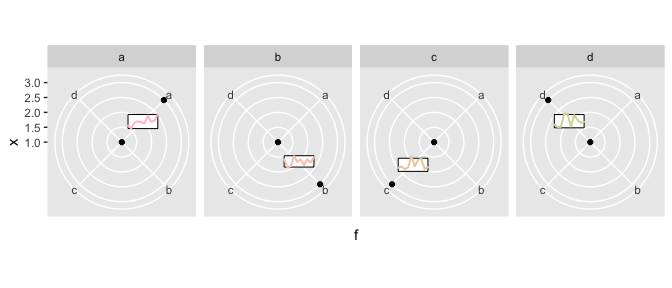# Overview of the egg package

#### 2019-07-13

This document provides a brief overview of the main utiilty functions included in the egg package.

## Exposing ggplot2 layouts

The function expose_layout can be useful to illustrate the structure of ggplot2 plots, e.g. when ying to customise and/or post-process the gtable layout.

p1 <- qplot(mpg, wt, data = mtcars, colour = cyl)
p2 <- qplot(mpg, data = mtcars) + ggtitle("title")
p3 <- qplot(mpg, data = mtcars, geom = "dotplot")
p4 <-
p1 + facet_wrap( ~ carb, nrow = 1) + theme(legend.position = "none") +
ggtitle("facetted plot")
pl <- lapply(list(p1, p2, p3, p4), expose_layout, FALSE, FALSE)
grid.arrange(
grobs = pl,
widths = c(1.2, 1, 1),
layout_matrix = rbind(c(1, 2, 3),
c(4, 4, 4))
)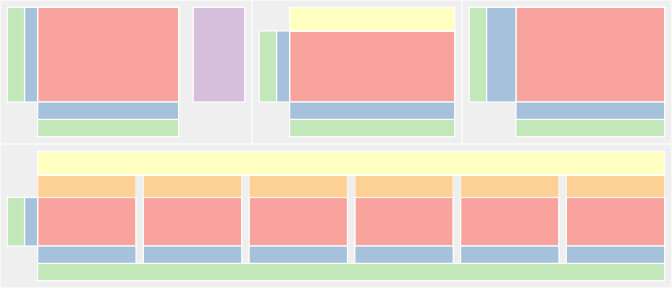## Setting panel size

In some cases, having ggplot2 expand the plot panel to best fit the available space isn’t ideal: for instance, we may want to produce multiple plots to appear on different slides of a presentation, and the successive pages should have the exact same layout for smooth visual transition. Another use-case is to embed multiple separate graphics in a drawing/page layout software. In this situation the plot alignement will be made manually, but the plots should not be rescaled (otherwise the fonts would be distorted). For such situations, the easiest solution is to set fixed dimensions to the gtable produced by ggplot2.

The function set_panel_size helps set the panel size (width, height) to absolute measurements in the form of grid units. In the case of a facetted plot, all panels are set to the same value.

  p1 <- qplot(mpg, wt, data = mtcars, colour = cyl)
p2 <- p1 + facet_wrap( ~ carb, nrow = 1)
grid.arrange(grobs = lapply(
list(p1, p2),
set_panel_size,
width = unit(2, "cm"),
height = unit(1, "in")
))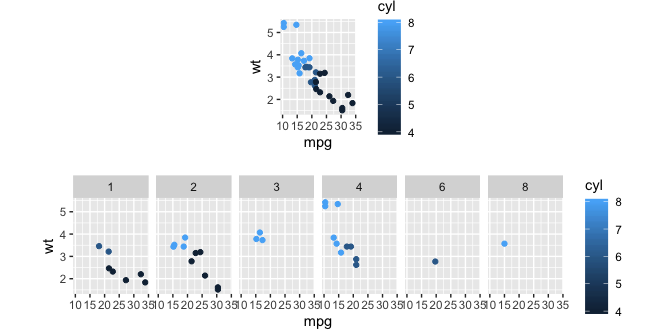Note that the total size is now fixed, therefore when exporting the plot on a device it can be useful to query the size and set the width and height accordingly, to avoid clipping or white margins. This extra step is enabled by default when saving the results to a file.

## Aligning complex ggplots

gridExtra::grid.arrange provides no way to align the panels of individual plots. While this is achievable with low-level gtable functions, it often requires substantial effort on a case-by-case basis. The egg package introduces a general strategy for such layout manipulations, with the following steps:

• decompose each plot into a 3x3 layout, where the central cell corresponds to the core panels, surrounded by axes, legends, etc.
• set the core width and height to a fixed dimension
• align the individual 3x3 gtables using rbind/cbind
p1 <- ggplot(mtcars, aes(mpg, wt, colour = factor(cyl))) +
geom_point()

p2 <- ggplot(mtcars, aes(mpg, wt, colour = factor(cyl))) +
geom_point() + facet_wrap(~ cyl, ncol = 2, scales = "free") +
guides(colour = "none") +
theme()

p3 <- ggplot(mtcars, aes(mpg, wt, colour = factor(cyl))) +
geom_point() + facet_grid(. ~ cyl, scales = "free")

g1 <- ggplotGrob(p1)
g2 <- ggplotGrob(p2)
g3 <- ggplotGrob(p3)

fg1 <- gtable_frame(g1, debug = TRUE)
fg2 <- gtable_frame(g2, debug = TRUE)
fg12 <-
gtable_frame(gtable_rbind(fg1, fg2),
width = unit(2, "null"),
height = unit(1, "null"))
fg3 <-
gtable_frame(
g3,
width = unit(1, "null"),
height = unit(1, "null"),
debug = TRUE
)
grid.newpage()
combined <- gtable_cbind(fg12, fg3)
grid.draw(combined)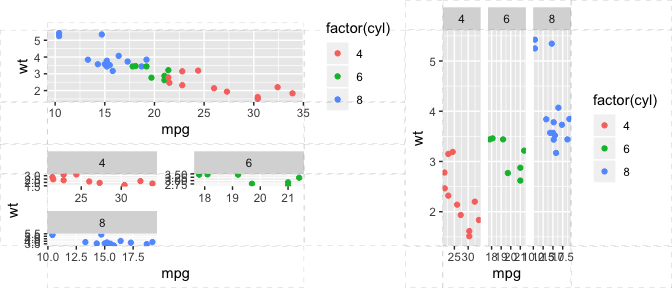## Arranging and aligning multiple plots

Using this generic strategy, we can easily align arbitrary plots (facetted or single-panel), with the convenience function ggarrange,

p1 <- ggplot(mtcars, aes(mpg, wt, colour = factor(cyl))) +
geom_point()+ theme_article() + theme(legend.position = 'top')
p2 <- ggplot(mtcars, aes(mpg, wt, colour = factor(cyl))) +
geom_point() + facet_wrap(~ cyl, ncol = 2, scales = "free") +
guides(colour = "none") +
theme_article()

ggarrange(p1, p2, widths = c(1.5,2))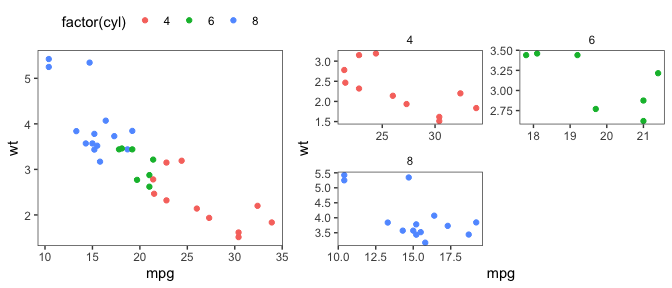Note that custom widths and heights may be provided for the layout.

p <- ggplot()
ggarrange(p, p, p, widths = c(3, 1), heights = c(5, 1))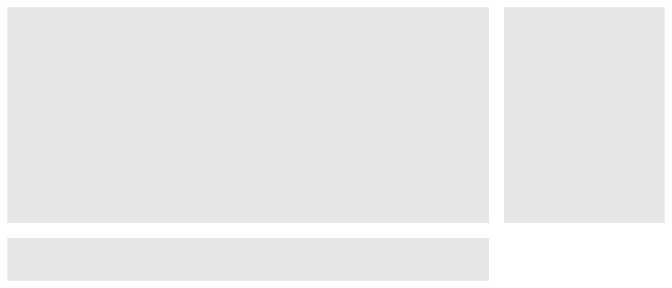For convenience, labels can be added to refer to the subfigures. All parameters of textGrob can be used for the formatting of the labels, including the positioning (x, hjust, etc.).

ggarrange(p1, p2, p3, ncol=2,
labels = c("A", "b)", "iii."),
label.args = list(gp=gpar(font=4), x=unit(1,"line"), hjust=0))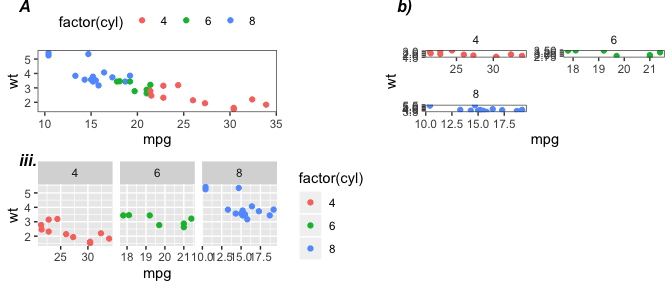The package provides two functions for labelling facetted plots in a more compact manner, removing panel strips and using in-panel tags instead:

d = data.frame(
x = 1:90,
y = rnorm(90),
red = rep(letters[1:3], 30),
blue = c(rep(1, 30), rep(2, 30), rep(3, 30)))

p <- ggplot(d) +
geom_point(aes(x = x, y = y)) +
facet_grid(red ~ blue)

tag_facet(p)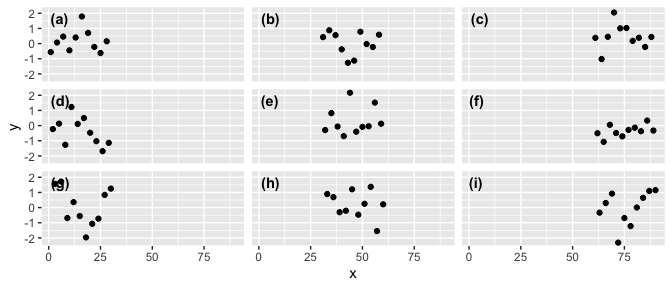tag_facet_outside(p)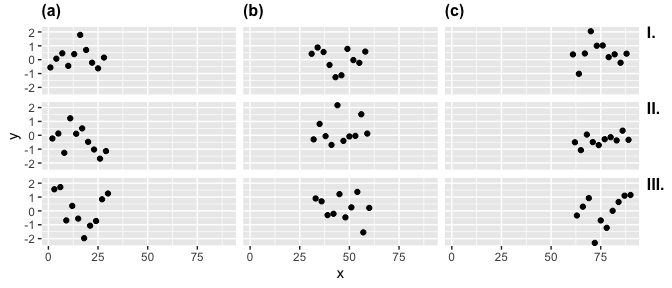## Custom themes

egg provides theme_article and theme_presentation,

d = data.frame(
x = 1:90,
y = rnorm(90),
red = rep(letters[1:3], 30),
blue = c(rep(1, 30), rep(2, 30), rep(3, 30)))

p <- ggplot(d) +
geom_point(aes(x = x, y = y)) +
facet_grid(red ~ blue)

p + theme_article()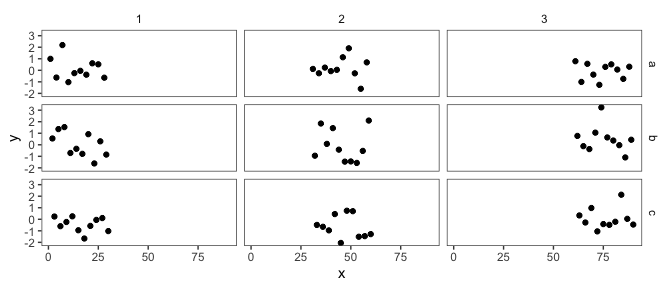## Symmetric axis scale

The function symmetric_range helps align the 0 value of adjacent panels in facetted plots with asymmetric range of data in each group.

df = data.frame(x = c(1, 2),
y = c(5, 0.2),
group = c(1, 2))
p <- ggplot(df, aes(x = x, y = y)) +
geom_point() +
facet_wrap( ~ group, scale =
"free")
p + scale_y_continuous(limits = symmetric_range)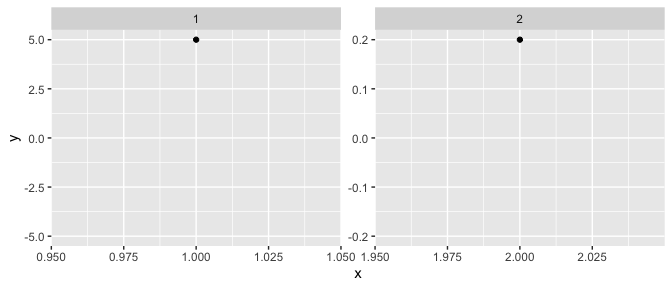## Custom geom

The function geom_custom extends the ggplot2 function annotation_custom to cases where multiple grobs are to be placed, e.g. on different panels, or at different positions in a plot. This geom is a bit special in that it does not truly respect a grammar of graphics – arbitrary grobs can be plotted, with no explicit mapping to variables. Its typical use would be to place annotations (images, tables, …). The data used to create the annotation is passed as a list-column.

codes <- data.frame(country = c("nz","ca","ar","fr","gb","es"))
codes$y <- runif(nrow(codes)) gl <- lapply(codes$country,
paste0(.x,".png"),
package="egg")))

codes$raster <- I(gl) ggplot(codes, aes(x = country, y = y)) + geom_point() + geom_custom(data = codes, aes(data=raster), grob_fun = rasterGrob, fun_params = list(height=unit(1,"cm"))) + scale_y_continuous(breaks=NULL, "") + theme(panel.grid = element_blank())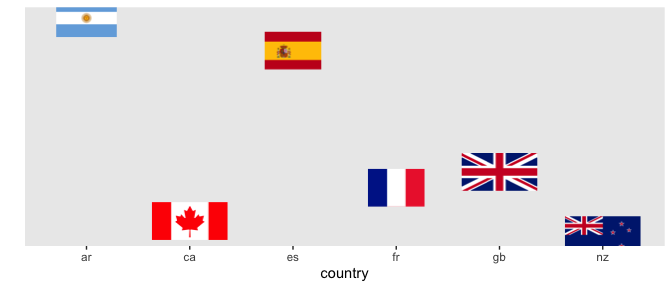The list-column format allows passing grobs directly, in which case the grob_fun function should be identity, codes$raster <- I(lapply(codes$raster, function(x) rasterGrob(x, height=unit(1,"cm")))) ggplot(codes, aes(x = country, y = y)) + geom_point() + geom_custom(data = codes, aes(data=raster), grob_fun = identity)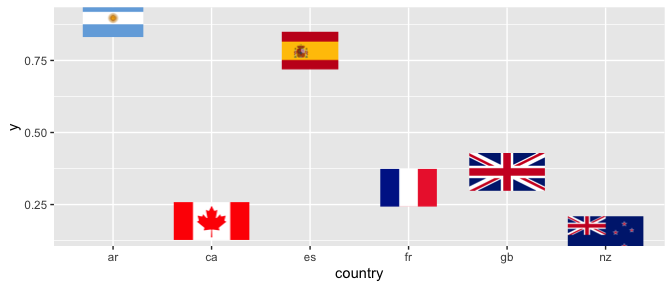Note that such grobs need to have x and y slots, which will be mapped to the appropriate location. It is therefore often necessary to create a wrapper with such fields, as illustrated below. Because the grobs are manually “mapped”, independently of the main ggplot, this geom also allows the placing of arbitrary annotations without interference from transformed coordinate systems, etc. custom_grob <- function(data, x=0.5,y=0.5){ grob(data=data,x=x,y=y, cl="custom") } preDrawDetails.custom <- function(x){ pushViewport(viewport(x=x$x,y=x$y)) } postDrawDetails.custom <- function(x){ upViewport() } drawDetails.custom <- function(x, recording=FALSE, ...){ grid.rect(mean(x$data$x), mean(x$data$y), width=diff(range(x$data$x)), height=diff(range(x$data$y))) grid.lines(x$data$x, x$data$y, gp=gpar(col=x$data$col,lwd=2), default.units = "native") } d <- data.frame(x=rep(1:3, 4), f=rep(letters[1:4], each=3)) gl <- lapply(1:4, function(ii){ data.frame(x=seq(0.4,0.6,length=10), y = runif(10,0.45,0.55), col = hcl(h = seq(0,300,length=nrow(d)))[ii], stringsAsFactors = FALSE) }) subplots <- data.frame(f=letters[1:4], data = I(gl)) str(subplots) ## 'data.frame': 4 obs. of 2 variables: ##$ f   : Factor w/ 4 levels "a","b","c","d": 1 2 3 4
##  $data:List of 4 ## ..$ :'data.frame': 10 obs. of  3 variables:
##   .. ..$x : num 0.4 0.422 0.444 0.467 0.489 ... ## .. ..$ y  : num  0.469 0.452 0.491 0.498 0.492 ...
##   .. ..$col: chr "#FFC5D0" "#FFC5D0" "#FFC5D0" "#FFC5D0" ... ## ..$ :'data.frame': 10 obs. of  3 variables:
##   .. ..$x : num 0.4 0.422 0.444 0.467 0.489 ... ## .. ..$ y  : num  0.527 0.471 0.481 0.547 0.508 ...
##   .. ..$col: chr "#FACABC" "#FACABC" "#FACABC" "#FACABC" ... ## ..$ :'data.frame': 10 obs. of  3 variables:
##   .. ..$x : num 0.4 0.422 0.444 0.467 0.489 ... ## .. ..$ y  : num  0.469 0.478 0.459 0.471 0.548 ...
##   .. ..$col: chr "#EDD0AD" "#EDD0AD" "#EDD0AD" "#EDD0AD" ... ## ..$ :'data.frame': 10 obs. of  3 variables:
##   .. ..$x : num 0.4 0.422 0.444 0.467 0.489 ... ## .. ..$ y  : num  0.477 0.46 0.462 0.549 0.549 ...
##   ..- attr(*, "class")= chr "AsIs"
ggplot(d, aes(f,x)) +
grob_fun = custom_grob)